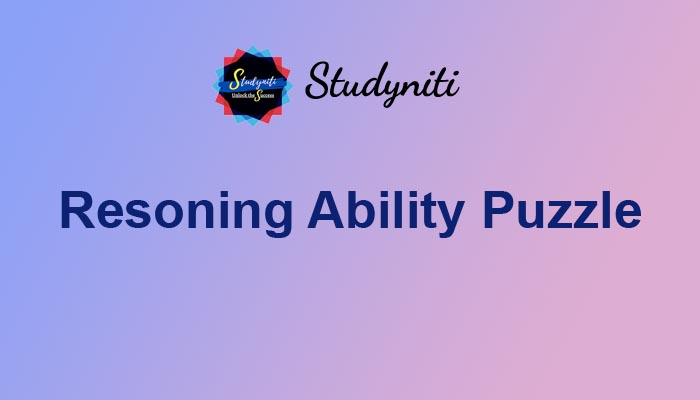## Payment Processing...# Reasoning Ability Puzzle - Sep 24 2020

Here we are providing new series of Reasoning Questions for upcoming exams, so the aspirants can practice it on a daily basis.

Direction (1-5): Study the following information carefully and answer the below questions.

Eight persons-L, M, N, O, P, Q, R, and S are sitting around a circular table. Some of them are facing inside and some of them are facing outside. Not more than two persons are facing the same direction sit together. All the information is not necessarily in the same order.

P and Q are sitting opposite to each other. Q is neither an immediate neighbor of N nor L. N sits second to the left of M, both are facing the opposite direction. O and Q are immediate neighbors. Only one person sits between L and N. Q and S are facing the same direction but opposite to L. Immediate neighbors of N are facing the opposite direction. The number of persons sits between P and S is the same as the number of persons sits Q and R, who sits immediate left of N. Immediate neighbors of M are facing the same direction. R is facing towards the center.

1) Who among the following person sits second to the right of Q?

A.M

B.N

C.P

D.L

E.R

2) How many people sit between P and O?

A.Two

B.Three

C.Four

D.Either a or c

E.None

3) Which among the following statement(s) is/are True?

I). S and L are immediate neighbours

II). No one sits between Q and O

III). R and M are facing the opposite direction

A.Only I and III

B.Only III

C.Only I and II

D.Only II

E.All I, II and III

4) What is the position of N with respect to L?

A.Second to the right

B.Third to the right

C.Second to the left

D.Third to the left

E.both a and d

5) Four of the following five are similar in such a way to form a group, who among the following doesn’t belong to the group?

A.S

B.N

C.R

D.Q

E.O

Explanation

N sits second to the left of M, both are facing the opposite direction.

Only one person sits between L and N.

From the above conditions, there are two possibilities.P and Q are sitting opposite to each other.

Q is neither an immediate neighbor of N nor L.

O and Q are immediate neighborsThe number of persons between P and S is the same as that of Q and R, who sits immediate left of N.Immediate neighbors of M are facing the same direction.

Not more than two persons are facing the same direction sit together.

Immediate neighbors of N are facing the opposite direction.

Q and S are facing the same direction but opposite to L.R is facing towards the center.

From the above condition, case1 was dropped. Case2 shows the final arrangement.1) Answer: E

2) Answer: D

3) Answer: E

4) Answer: A

5) Answer: E